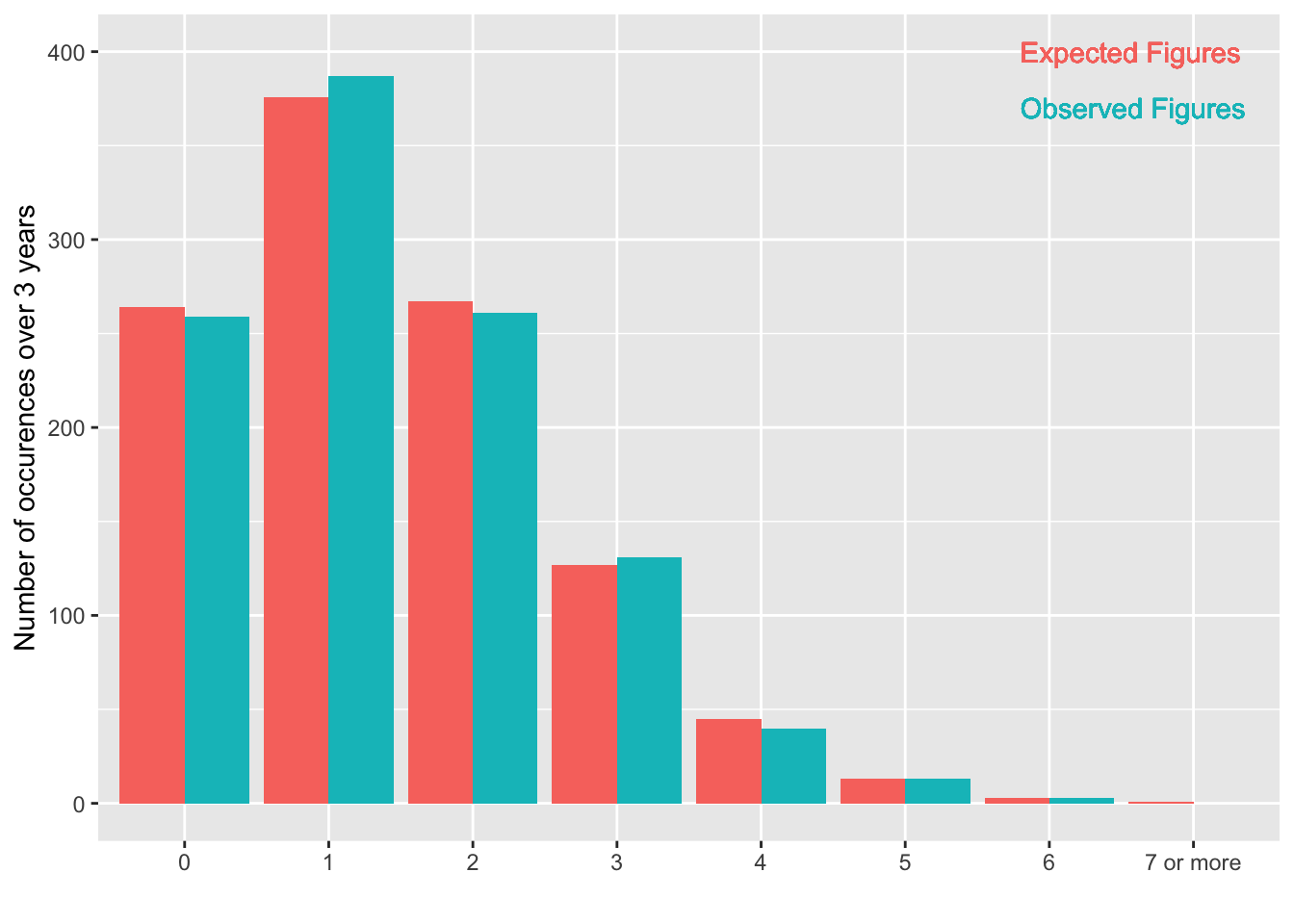Data are from Office for National Statistcs Homicide data, 2017

library(ggplot2)
#attach(df)
p <- ggplot(df, aes(x= dailyNumberOfRecordedHomicideIncidents)) # constructs initial plot object, p

#colour palette failing on the bars so omitted
#p <- p + scale_colour_brewer(palette = "Accent") # sets the colour palette

p <- p + geom_bar(aes(y=Occurences,fill=FiguresType),stat = "identity",position="dodge") # assigns bar-chart type with side by side (dodged) bars
p <- p + geom_text(aes(x = 6.8, y = 370, label = "Observed Figures", color = "ObservedFigures", hjust=0)) # our custom legend
p <- p + geom_text(aes(x = 6.8, y = 400, label = "Expected Figures", color = "ExpectedFigures", hjust=0)) # our custom legend
p <- p + theme(legend.position="none") # removes the default legend
p <- p +  labs(x="", y="Number of occurences over 3 years")
pFigure 8.5 Observed and expected (assuming a Poisson distribution) daily number of recorded homicide incidents, 2014 to 2016, England and Wales (ONS data)

Table 10.3 (page 270-272) Hypothesis test of Poisson distribution

n =  0:7
observed=df$Occurences[df$FiguresType=="ObservedFigures"]
homicides = n*observed
mean = sum(homicides)/1095 #  average number per day
mean
##  1.410959
p=dpois(0:5,mean) # poisson probability up to 5
p=1-sum(p[1:6])  # probability of 6 or more
p   # probablities for 0 to 6 or more
##  0.243909285 0.344145978 0.242787916 0.114187924 0.040278617 0.011366295
##  0.003323985
expected=p*1095
expected # does not match ONS fgures exactly
##  267.080667 376.839846 265.852768 125.035777  44.105086  12.446093
##    3.639764
chisq.test(observed[-8],p=p) # remove final 0 for 7 or more
## Warning in chisq.test(observed[-8], p = p): Chi-squared approximation may
## be incorrect
##
##  Chi-squared test for given probabilities
##
## data:  observed[-8]
## X-squared = 1.4111, df = 6, p-value = 0.9652